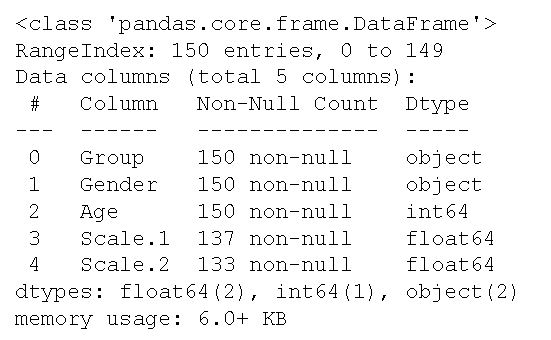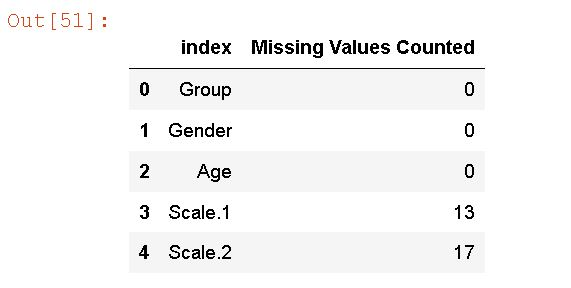##### How to Prepare Data for Analysis in Python with Pandas

In this tutorial, I will walk you through some of the steps that you can use to prepare your data for statistical analysis.

## Why Prepare Data?

In some cases, your data will be perfectly fine for data analysis and you may not need to do anything to prepare it for data analysis. However, in most caes, your data will have errors, bad variable names, missing values, or other things that will make data analysis a bit rough.

## Requirements

To follow this post you need to have Python and Pandas installed. Pandas can be installed using pip: `pip install pandas`. Of course, you can install a Scientific Python distribution such as Anaconda and get both Python and Pandas, among other great packages, installed in a go!

## Importing Data from a CSV File in Python with Pandas

Pandas can be used to read data from many different file formats. Here's how to read a CSV file with Pandas:

``````import pandas as pd

Check out the excellent blog https://www.marsja.se for a lot of great Python and Pandas tutorials. Many of the things I have added to this tutorial, are things I have learned by the guides and how-tos there. Make sure to check it out.

### Exploring the Data

Pandas has a range of different useful methods to explore the data. For example, we can explore the dimensions of the dataset using shape:

``````df.shape

# Output: (149, 5)``````

This gives us the information that there are 149rows

If we, on the other hand, want to get information about the dataset we can use the info() method:

``df.info()``Here we get some important information about the dataset. For example, we can se that there are were no column names in the dataset. Although, it is possible to change, or assign, column names
after we have read the data, the next step, here, is to read the data again and set the column names using the names parameter:

``````column_names = ["Group", "Gender", "Age",
"Scale.1", "Scale.2"]

names=column_names)``````

Now, we can get the column names by printing them. df.columns will do the trick!

## Assigning variable names

If we, on the other hand, wanted to change the column names we can do this in a number of ways. Here's how to do this:

``````df.columns = ["Treatment", "Sex", "Age",
"Depression", "Anxiety"]``````

We can also get the column names from the Pandas dataframe using df.columns. In the next section, we are going to deal with missing values.

## Dealing with missing values (NA)

If the number of missing values is small, we may safely drop those rows. However, we should take care: a
few missing values in this variable and a few missing values in that variable can quickly add up to a lot of
incomplete data. As we saw, in the output of the info() method there are missing values in the two last columns.

### Counting Missing Values

Here's how to count missing values across all variables (i.e., columns) in the Pandas dataframe:

``df.isnull().sum().reset_index(name='Missing Values Counted')``

In the output we see that there are 0 missing values in the three first columns: "Group", "Gender", and "Age". However, there are 13 and 17 missing values, in the "Scale.1" and "Scale.2" columns, respectively.
In the next section, we are going to drop the rows containing missing values.

### Dropping Missing Values

Here's how to drop missing values from Pandas dataframe:

``````df_complete = df.dropna()
df_complete.shape
# Output: (121, 5)``````

Again, using shape we can see that we have dropped a number of rows from the dataframe. To delete rows with at least one missing values we just used the dropna() method. We made a new (i.e., a copy) of the dataframe containing only complete cases.### Dropping Columns/Variables

Sometimes we may also want to delete columns from the dataframe! Here's how we'd drop the column named "Age":

``df.drop('Age', axis=1)``

Note, that we used the axis parameter and set it to "1". We did this because we wanted to drop a column! If we, on the other hand, wanted to drop a row we don't have to use this argument. Finally, if you want to drop multiple columns from the dataframe add a list with the column names!
Of course, dropping columns in the example data is not actually needed.

That was it, for this time! There are, of course, plenty of other things you may want to do when preparing your data for data analysis! For example, you may need to clean your data, create dummy variables (if doing regression analysis) of categorical variables and so on.

## Conclusion

In this post, you have learned some data preparation methods in Python. You have learned how to read data from a CSV file, explore the data some, and how to see how many missing values there are and how to drop them. Also, you have learned about column names and how to set them.
Finally, you also learned how to drop columns.

You are viewing a single comment. View All
PsyPy (2)

Thanks for this. Very insightful post.